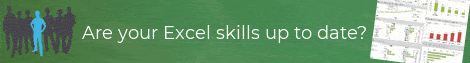## Tuesday, 30 July 2013

### Excel Tip - The NETWORKDAYS function

Before we get into today's post, just a little reminder that tomorrow (31st July) is your last chance to get the 20% discount on Mynda Treacy's Excel Dashboards course. Also, don't forget I'll be sending an absolutely free copy of my Introduction to Pivot Tables course to everyone who signs up to Mynda's course.

This week's post is a quick one on a useful function that allows you to calculate the total number of work days between two dates. This is particularly useful for budgeting, where production (or sales) is driven by the number of working days in each period.

The syntax of the NETWORKDAYS function is as follows:

=NETWORKDAYS(Start date,End date,Holidays)

The Holidays variable is optional, so at its most basic the function is as follows:

=NETWORKDAYS(Start date,End date)

The dates need to be either entered as their serial numbers (see my earlier post on elapsed time in Excel that explains how Excel dates work), or refer to a cell that includes the date (usually more what you would want, rather than hard-coding a date in the formula.

If you do need to hard-code the date in the formula, use the DATE function which returns the serial number for a date.

The DATE function is used in the format =DATE(Year,Month,Day), so =DATE(2013,7,30) returns the serial number for today's date (30th July 2013) which, incidentally, is 41485.

Taking the more useful approach where the dates are held in cells, let's assume that we have our start date in cell A1 and our end date in cell A2, then:

=NETWORKDAYS(A1,A2)

will give us the number of working days between the two days (INCLUDING the start and end dates).

So, if A1=1/7/2013 and A2=31/7/2013, then:

=NETWORKDAYS(A1,A2)

returns 23, which is 31 days less 4 Saturdays and 4 Sundays.

This is great, but what if we are in the US where 4th July is a holiday?

To allow for holidays, we allocate a range to enter holiday dates. So let's say we decide to use the range B1:B20 to enter the holiday dates for the year and put 4/7/2013 in that list (along with any other holidays we want to allow for).

Now if we use the formula:

=NETWORKDAYS(A1,A2,B1:B20)

this time we get 22 (as long as 4/7/2013 is the only July date in the range B1:B20).

I hope you find it useful...If you enjoyed this post, go to the top of the blog, where you can subscribe for regular updates and get your free report "The 5 Excel features that you NEED to know".

1.1.